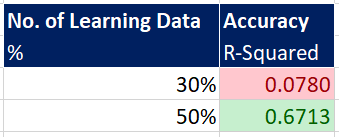top of page
Search

# How To Verify Your AiSara Model

We know how to learn and predict with AiSara. Sometimes, a question comes to mind when we predict with AiSara: “How do I verify my model with AiSara? How do I know if the prediction is reliable/accurate?”. Within this article, we will shine some light regarding this matter and we hope it will help you in validating your model with AiSara.

## Walkthrough

Before we begin, I will briefly explain regarding the workflow. These are the steps that we will discuss:

1. Prepare your training and testing dataset

2. Use AiSara to Learn from the training data, then predict with the test data.

3. Calculate the Pearson’s Correlation (R2) between the true value, and the predicted value of AiSara.

4. Repeat step 1 and 3, but with a higher number of training data set.

5. Compare the results (by using R-Squared, R2) of AiSara between the 2 models.

We have automated this for you, with the Blind Test function in our Excel, we’ll talk more about it in the end.

We will be using a sample oil and gas History Matching dataset, which consists of 6 variables to find a minimum target value which represents the minimum error, also known as hm_error in the dataset. Minimum error means, the best solution possible. In case you are wondering where the dataset comes from, it is from hours of simulation to produce the results as shown. Now let us proceed to the first step which is:

Let’s set aside some dataset for training and testing

What is training dataset? A set of data that is used for learning.
What is testing dataset? A set of data that is independent of training dataset, to assess the performance of the trained model.The 30% of dataset used for training

So firstly, we will set aside 30% of the data for training and 70% of the data for testing. There are 6 input variables as shown above, and 1 output variable, which is hm_error.Predicting with the testing dataset

Then we learn the 6 inputs with the training data and predict the hm_error with testing data.Calculating the Pearson's Correlation between the True Value of hm_error against AiSara's Predicted Value of hm_error.

Once we have predicted the testing data with our trained model, here is where we verify our model using the Pearson correlation, which you can learn more about it here. We can simply use the RSQ function in excel, to find our R2. For this walkthrough, the function we used is “=RSQ(True Output, Predicted Output)”, and the result is R2 0.0780. The low R2 result shows that there is almost no linear correlation between the true output value with the predicted output value, which means the model is weak.

In Summary, the closer the R2 is to 1, the higher the correlation between AiSara’s prediction model and the minimum history match error.We can also plot the True Output hm_error vs. Predict Output hm_error to have a quick glance if any of there are any correlation exists.

Great, we have our first test. Next, we repeat the same process as above by using 50% training dataset and 50% testing data. Let’s cut to the chase, and here are the results

## ResultsThe R2 of a model that was trained by 30% data and 50% data respectivelyNotice the Pearson Correlation plot, the more quality data that is used for learning, the closer the blue dots are to the line, signifying a better correlation and accuracy of AiSara’s model towards the minimum history matching error.

It may take a while to run this process manually, that is why we have automated this process for you by using the Blind Test function. It’s easy to use, just select your learned cell and specify the output that you would like to run the blind test on and let excel do all the work for you. Below, is the result of the blind test:You can find the Blind Test button hereThe Blind Test - We have automated the process of verifying your model for you. X-axis shows the percentage of training dataset used for the model, whereas the y-axis shows the value of R2 based on the testing data of the model.

Thank you for taking the time to read this article, we hope this article can be of benefit to you. We have shared with you the methodology in verifying an AiSara model, and the same methodology can be used for other machine learning/artificial intelligence models.

We are here to help, thus if you have any questions or if you would like to give AiSara a try, we would be more than happy to assist you. Do contact us at enquiry@aisara.ai.